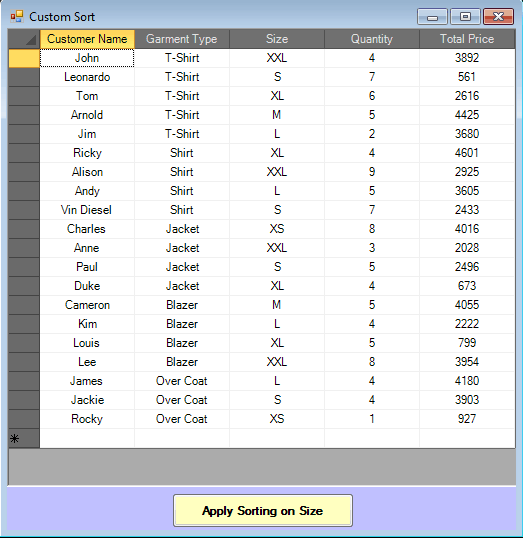The blazing fast .NET grid, C1FlexGrid in the WinForms Edition of ComponentOne Studio provides a number of ways to add flexibility to your applications. Sorting is one of the most useful operations that users want to perform, when it comes to grids. C1FlexGrid has built-in support for sorting with several options (ignore case, use the displayed value, etc). It provides a Sort method which can be directly used to sort the contents of the grid based on a column. If you need more flexibility, you need to create a class that implements the IComparer.aspx) interface. Basically, a comparison method is made for simple comparison operations that are not complicated enough for reusability to be of any concern, e.g. sorting a list of customers by their first name. This is a simple operation, hence it does not need additional data.

### User Story: Sorting Sizes of Garments

Consider the example where, there is no notion of 'larger' and 'smaller', e.g. sizes available for a particular garment (XS, S, M, L, XL and XXL) in a store. You might want to compare those sizes and display the ordered data in the grid. That can be complicated, and a separate comparer should be used for this purpose.### IComparer Interface

The System.Collections.IComparer interface has a single method called System.Collections.IComparer.Compare(System.Object,System.Object) that takes two objects as arguments (in this case, they will be Row objects) and returns -1, 0, or +1. This interface is used in conjunction with the Sort method and provides a way to customize the sort order of a collection. The advantage of using IComparer is that you can create a number of sorting options, and you have the option of passing more specific and more appropriate sorting criteria to the Sort method. IComparer allows you to define re-usable, well-encapsulated comparers. This is especially useful if the comparison needs to know some additional information.

### Applying Custom Sort on C1FlexGrid

Among the five overloads of the Sort method in C1FlexGrid, two accept an object of IComparer interface as one of their arguments and hence, can be used to sort a group of rows using the specified comparer. In order to apply sorting based on the sizes (XS, S, M, L, XL and XXL) available for the garments, implement IComparer interface in a class. This class will be a ‘comparer’ and will impose the sorting order. To sort the grid using IComparer, create a new instance of the class that implements it and pass that instance to the Sort method of the grid.

``````
private void sort_Click(object sender, EventArgs e)
{
c1FlexGrid1.Sort(new MyComparer(c1FlexGrid1)); // Perform a custom sort on the grid.
}

public class MyComparer: IComparer
{
C1FlexGrid cfg;
public MyComparer(C1FlexGrid cfg)
{
this.cfg = cfg;
}
public int Compare(object r1,object r2)
{
int cmp = 0;
string s1 = cfg[((Row)r1).Index, 3].ToString();
string s2 = cfg[((Row)r2).Index, 3].ToString();
if(s1=="XS")
{
if (s2 == "XS")
cmp = 0;
else
cmp = -1;
}
else if (s1 == "XXL")
{
if (s2 == "XXL")
cmp = 0;
else
cmp = 1;
}
else if (s1 == "S")
{
if (s2 == "XS")
cmp = 1;
else if (s2 == "S")
cmp = 0;
else
cmp = -1;
}
else if (s1 == "L")
{
if (s2 == "XS")
cmp = 1;
else if (s2 == "S")
cmp = 1;
else if (s2 == "M")
cmp = 1;
else if (s2 == "L")
cmp = 0;
else
cmp = -1;
}
else if (s1 == "M")
{
if (s2 == "XS")
cmp = 1;
else if (s2 == "S")
cmp = 1;
else if (s2 == "M")
cmp = 0;
else
cmp = -1;
}
else if (s1 == "XL")
{
if (s2 == "XXL")
cmp = -1;
else if (s2 == "XL")
cmp = 0;
else
cmp = 1;
}
return cmp;
}
}

``````